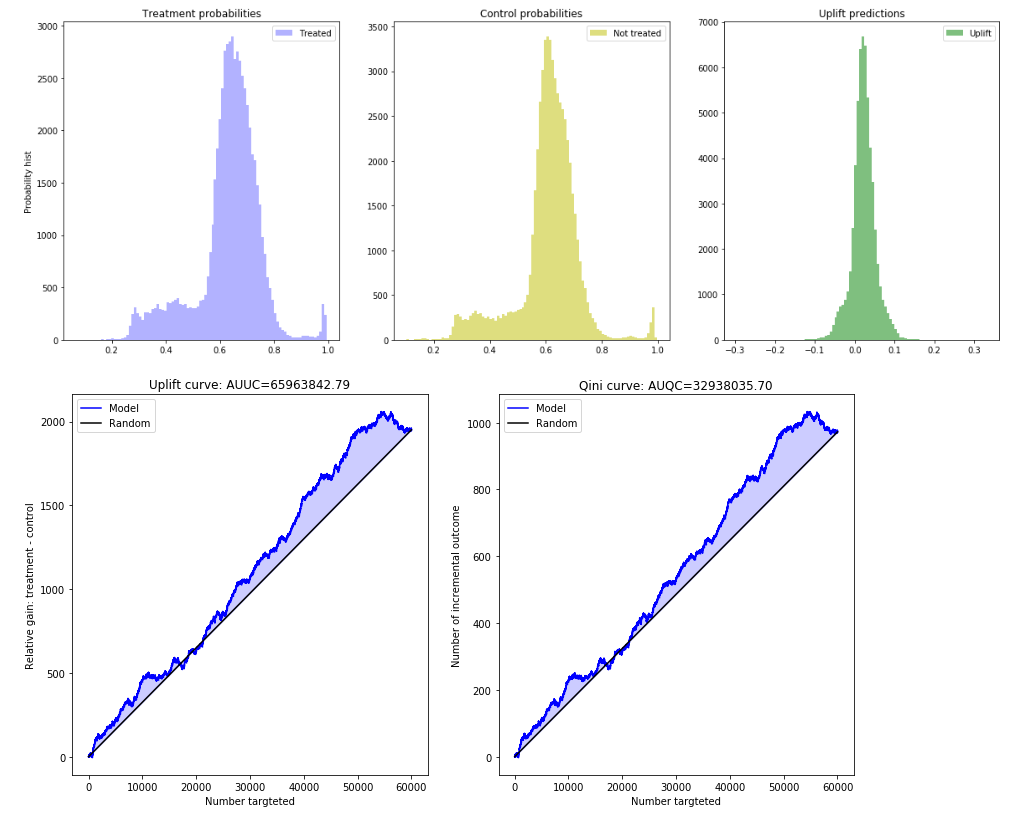# Quick Start¶

See the RetailHero tutorial notebook (EN, RU) for details.

Train and predict your uplift model

# import approaches
from sklift.models import SoloModel, ClassTransformation, TwoModels
# import any estimator adheres to scikit-learn conventions.
from catboost import CatBoostClassifier

# define approach
sm = SoloModel(CatBoostClassifier(verbose=100, random_state=777))
# fit model
sm = sm.fit(X_train, y_train, treat_train, estimator_fit_params={{'plot': True})

# predict uplift
uplift_sm = sm.predict(X_val)


Evaluate your uplift model

# import metrics to evaluate your model
from sklift.metrics import qini_auc_score, uplift_auc_score, uplift_at_k
# Uplift@30%
sm_uplift_at_k = uplift_at_k(y_true=y_val, uplift=uplift_sm, treatment=treat_val, k=0.3)
# Area Under Qini Curve
sm_qini_auc_score = qini_auc_score(y_true=y_val, uplift=uplift_sm, treatment=treat_val)
# Area Under Uplift Curve
sm_uplift_auc_score = uplift_auc_score(y_true=y_val, uplift=uplift_sm, treatment=treat_val)


Vizualize the results

# import vizualisation tools
from sklift.viz import plot_uplift_preds, plot_uplift_qini_curves

# get conditional predictions (probabilities) of performing a target action
# with interaction for each object
sm_trmnt_preds = sm.trmnt_preds_
# get conditional predictions (probabilities) of performing a target action
# without interaction for each object
sm_ctrl_preds = sm.ctrl_preds_

# draw probability distributions and their difference (uplift)
plot_uplift_preds(trmnt_preds=sm_trmnt_preds, ctrl_preds=sm_ctrl_preds);
# draw Uplift and Qini curves
plot_uplift_qini_curves(y_true=y_val, uplift=uplift_sm, treatment=treat_val);